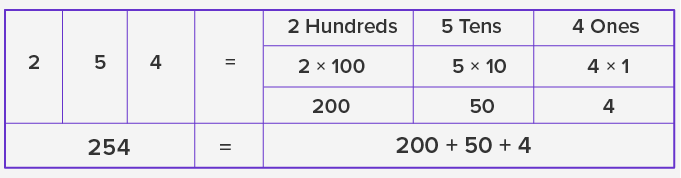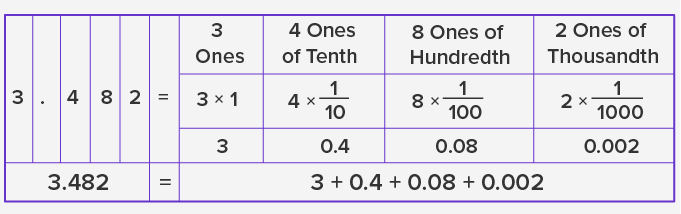Expanded Form With Decimals - Definition with Examples

The Complete K-5 Math Learning Program Built for Your Child

• 30 Million Kids

Loved by kids and parent worldwide

• 50,000 Schools

Trusted by teachers across schools

• Comprehensive Curriculum

Aligned to Common Core

Expanded Form with Decimals

Expanded form

The expanded form notation for the decimal numbers is the mathematical expression to show the sum of the values of each digit in the number. To write the expanded form of any number, let’s see how we use the place value system.

Place value system

The place value refers to the value of a digit in a number. The value for each number is computed using the position of number. Starting from right to left, we can understand the notations used in the place value using an example.

For the number 254, the place value chart is:Thus, the expanded form of the number 254 is 200 + 50 + 4.

Likewise, the expanded form of a number with a decimal or a fraction is written with a base 10-multiple denominator, represented by the power of 10.

For example, the number 3.482 in expanded form is written as:The expanded form of the number 3.482 is:

= 3 + 4108100 + 421000 ,or

= 3 + 0.4 + 0.08 + 0.002

Application

The scope of decimal numbers is in the areas where accuracy and precision are required. The concept of expanded form is useful in comprehending the value of a number as well as the numeric value of a quantity.

 Fun Facts The weights in the ratios of 1⁄20 , 1⁄10 , 1⁄5 , 1⁄2 and multiples of 10 were used in Indus valley civilization for the trade purpose. Rod calculus in ancient China used bamboo strips with the decimal system for math operations like multiplication during 305 BC. Mathematician Archimedes invented a decimal positional system for Sand Reckoner to represent large numbers which were multiples of 10.Won Numerous Awards & Honors